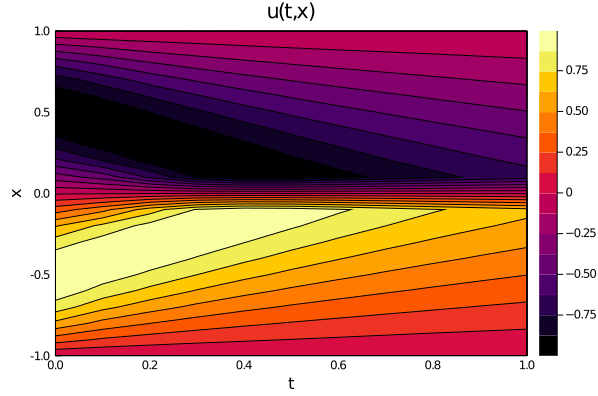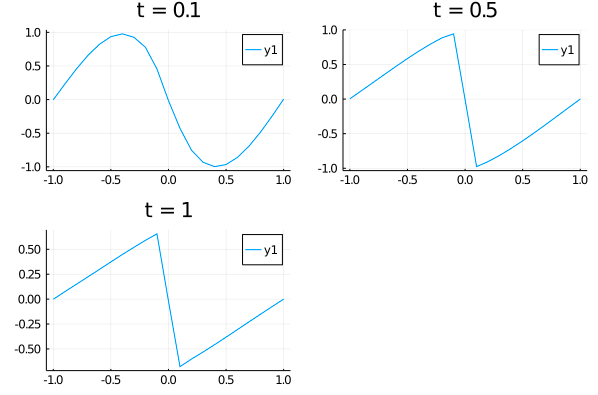# 1-D Burgers' Equation With Low-Level API

Let's consider the Burgers' equation:

$$$\begin{gather*} ∂_t u + u ∂_x u - (0.01 / \pi) ∂_x^2 u = 0 \, , \quad x \in [-1, 1], t \in [0, 1] \, , \\ u(0, x) = - \sin(\pi x) \, , \\ u(t, -1) = u(t, 1) = 0 \, , \end{gather*}$$$

with Physics-Informed Neural Networks. Here is an example of using the low-level API:

using NeuralPDE, Flux, ModelingToolkit, GalacticOptim, Optim, DiffEqFlux
import ModelingToolkit: Interval, infimum, supremum

@parameters t, x
@variables u(..)
Dt = Differential(t)
Dx = Differential(x)
Dxx = Differential(x)^2

#2D PDE
eq  = Dt(u(t,x)) + u(t,x)*Dx(u(t,x)) - (0.01/pi)*Dxx(u(t,x)) ~ 0

# Initial and boundary conditions
bcs = [u(0,x) ~ -sin(pi*x),
u(t,-1) ~ 0.,
u(t,1) ~ 0.,
u(t,-1) ~ u(t,1)]

# Space and time domains
domains = [t ∈ Interval(0.0,1.0),
x ∈ Interval(-1.0,1.0)]
# Discretization
dx = 0.05
# Neural network
chain = FastChain(FastDense(2,16,Flux.σ),FastDense(16,16,Flux.σ),FastDense(16,1))
initθ = Float64.(DiffEqFlux.initial_params(chain))
eltypeθ = eltype(initθ)
parameterless_type_θ = DiffEqBase.parameterless_type(initθ)
strategy = NeuralPDE.GridTraining(dx)

phi = NeuralPDE.get_phi(chain,parameterless_type_θ)
derivative = NeuralPDE.get_numeric_derivative()

indvars = [t,x]
depvars = [u]

_pde_loss_function = NeuralPDE.build_loss_function(eq,indvars,depvars,phi,derivative,nothing,
chain,initθ,strategy)

bc_indvars = NeuralPDE.get_variables(bcs,indvars,depvars)
_bc_loss_functions = [NeuralPDE.build_loss_function(bc,indvars,depvars,
phi,derivative,nothing,chain,initθ,strategy,
bc_indvars = bc_indvar) for (bc,bc_indvar) in zip(bcs,bc_indvars)]

train_sets = NeuralPDE.generate_training_sets(domains,dx,[eq],bcs,eltypeθ,indvars,depvars)
train_domain_set, train_bound_set = train_sets

pde_loss_function = NeuralPDE.get_loss_function(_pde_loss_function,
train_domain_set,
eltypeθ,parameterless_type_θ,
strategy)

bc_loss_functions = [NeuralPDE.get_loss_function(loss,set,
eltypeθ, parameterless_type_θ,
strategy) for (loss, set) in zip(_bc_loss_functions,train_bound_set)]

loss_functions = [pde_loss_function; bc_loss_functions]
loss_function__ = θ -> sum(map(l->l(θ) ,loss_functions))

function loss_function_(θ,p)
return loss_function__(θ)
end

f = OptimizationFunction(loss_function_, GalacticOptim.AutoZygote())
prob = GalacticOptim.OptimizationProblem(f, initθ)

cb_ = function (p,l)
println("loss: ", l , "losses: ", map(l -> l(p), loss_functions))
return false
end

# optimizer
opt = BFGS()
res = GalacticOptim.solve(prob, opt; cb = cb_, maxiters=2000)

And some analysis:

using Plots

ts,xs = [infimum(d.domain):dx:supremum(d.domain) for d in domains]
u_predict_contourf = reshape([first(phi([t,x],res.minimizer)) for t in ts for x in xs] ,length(xs),length(ts))
plot(ts, xs, u_predict_contourf, linetype=:contourf,title = "predict")

u_predict = [[first(phi([t,x],res.minimizer)) for x in xs] for t in ts ]
p1= plot(xs, u_predict,title = "t = 0.1");
p2= plot(xs, u_predict,title = "t = 0.5");
p3= plot(xs, u_predict[end],title = "t = 1");
plot(p1,p2,p3)See low-level API